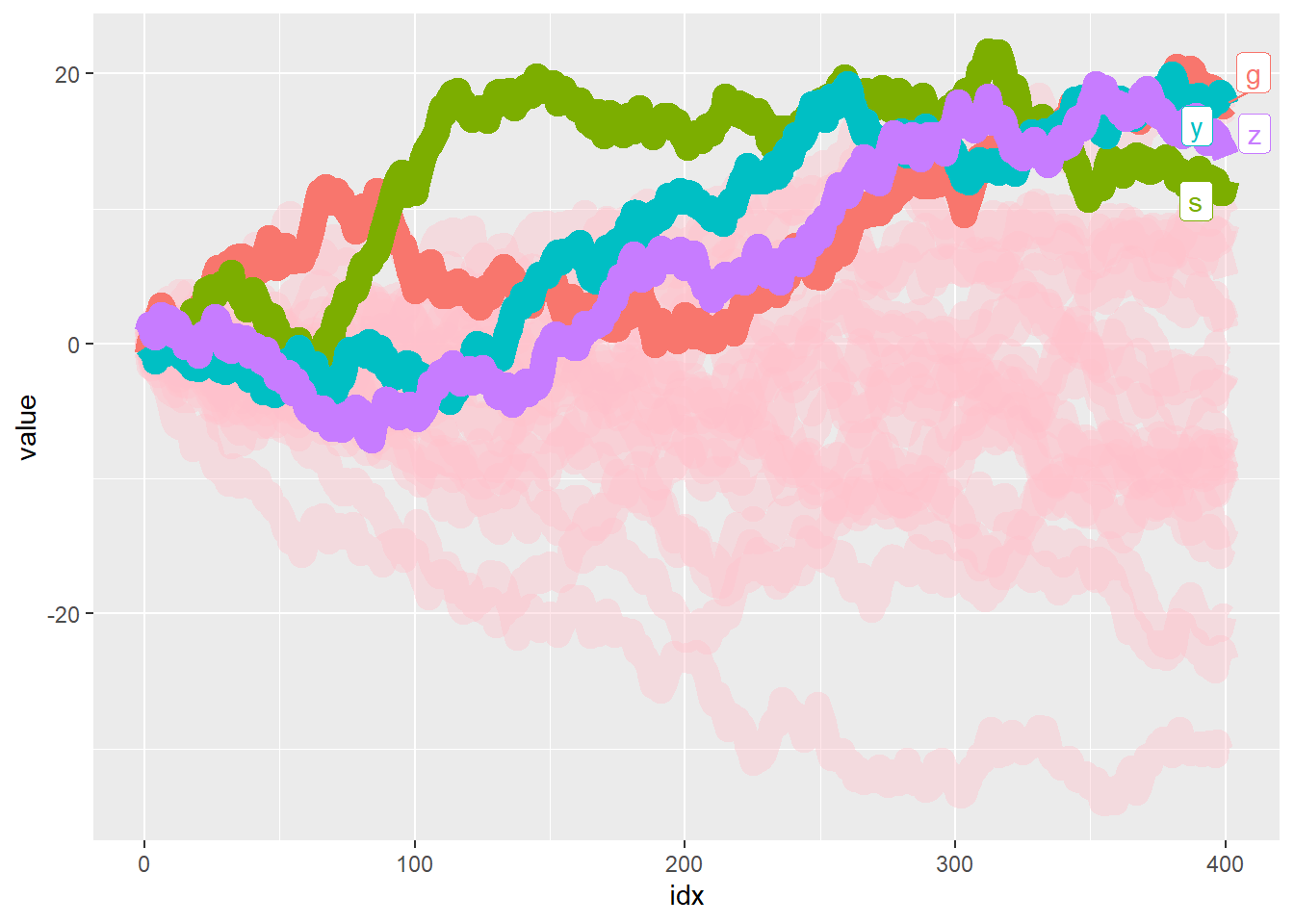# gghighlight 0.2.0

gghighlight 0.2.0 is released!

gghighlight
ggplot2
package
Author

Hiroaki Yutani

Published

February 17, 2020

gghighlight 0.2.0 is on CRAN a while ago. This post briefly introduces the three new features. For basic usages, please refer to “Introduction to gghighlight”.

## `keep_scales`

To put it simply, gghighlight doesn’t drop any data points but drops their colours. This means, while non-colour scales (e.g. `x`, `y` and `size`) are kept as they are, colour scales get shrinked. This might be inconvenient when we want to compare the original version and the highlighted version, or the multiple highlighted versions.

``library(gghighlight)``
``Loading required package: ggplot2``
``````library(patchwork)

set.seed(3)

d <- data.frame(
value = 1:9,
category = rep(c("a","b","c"), 3),
cont_var = runif(9),
stringsAsFactors = FALSE
)

p <- ggplot(d, aes(x = category, y = value, color = cont_var)) +
geom_point(size = 10) +
scale_colour_viridis_c()

p1 <- p + ggtitle("original")
p2 <- p +
gghighlight(dplyr::between(cont_var, 0.3, 0.7),
use_direct_label = FALSE) +
ggtitle("highlighted")``````
``````Warning: Tried to calculate with group_by(), but the calculation failed.
Falling back to ungrouped filter operation...``````
``p1 * p2``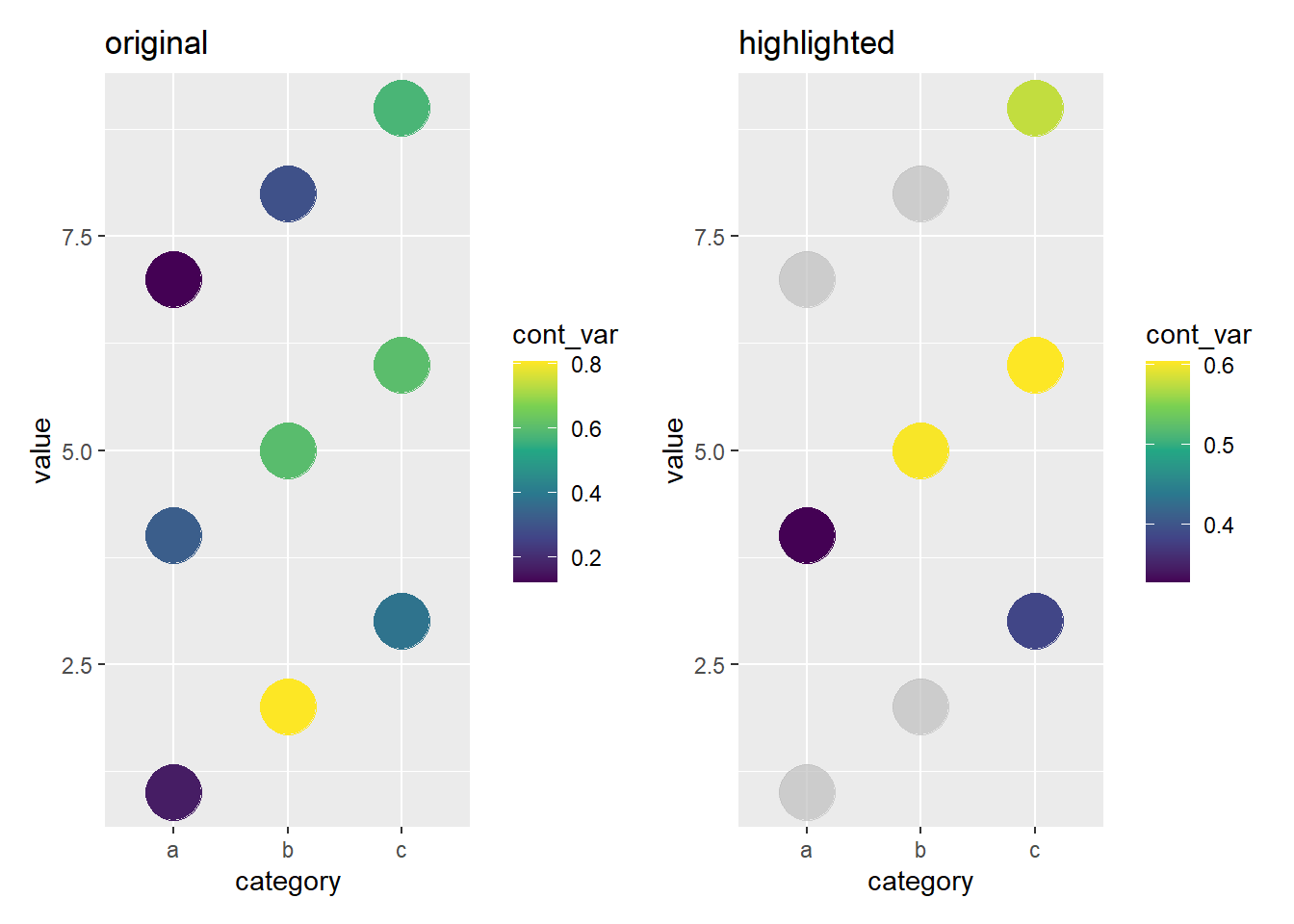You can see the colour of the points are different between the left plot and the right plot because the scale of the colours are different. In such a case, you can specify `keep_scale = TRUE` to keep the original scale (under the hood, gghighlight simply copies the original data to `geom_blank()`).

``````p3 <- p +
gghighlight(dplyr::between(cont_var, 0.3, 0.7),
keep_scales = TRUE,
use_direct_label = FALSE) +
ggtitle("highlighted (keep_scale = TRUE)")``````
``````Warning: Tried to calculate with group_by(), but the calculation failed.
Falling back to ungrouped filter operation...``````
``p1 * p3``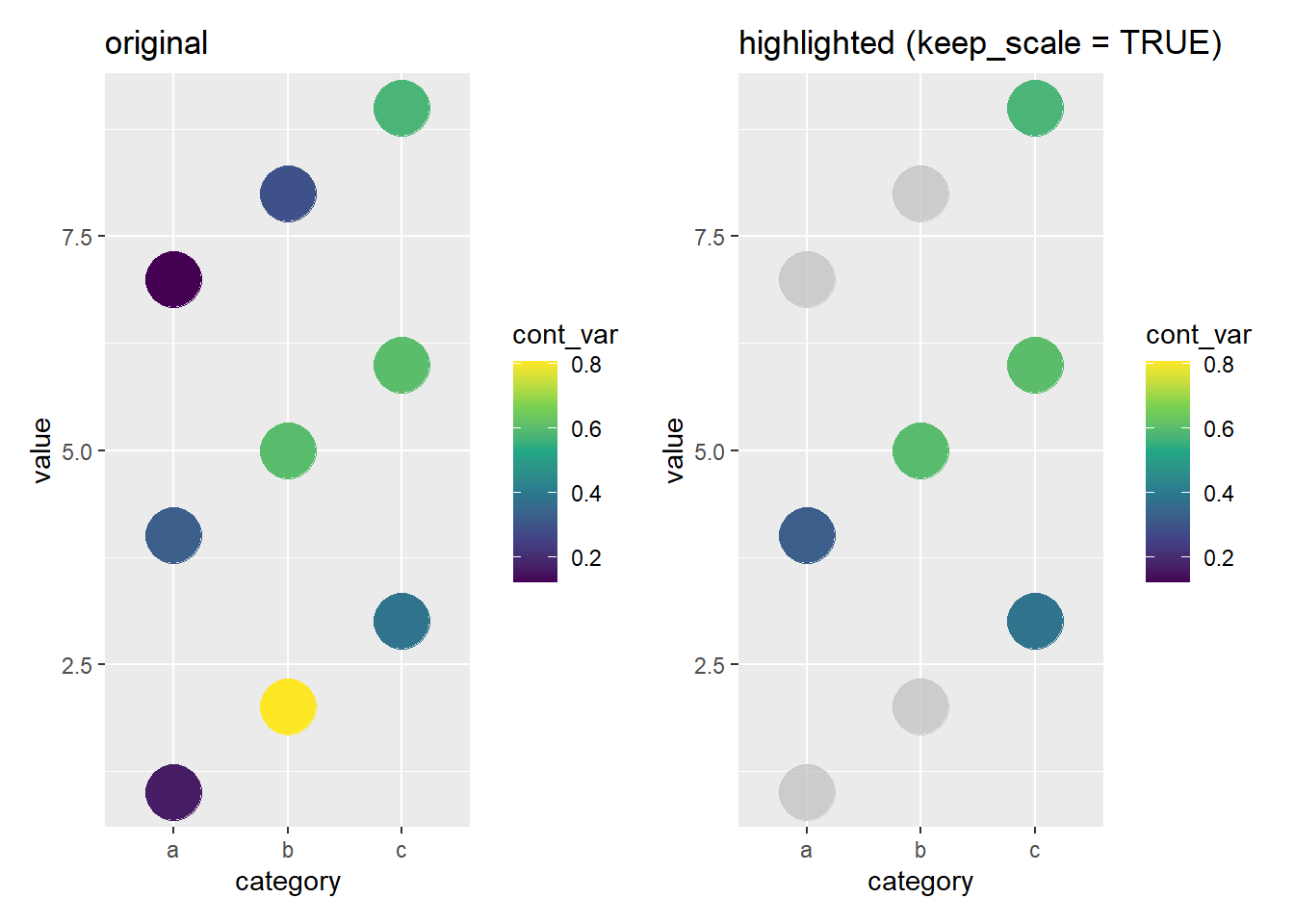## `calculate_per_facet`

When used with `facet_*()`, `gghighlight()` puts unhighlighted data on all facets and calculate the predicates on the whole data.

``Sys.setlocale(locale = "C")``
`` "C"``
``````set.seed(16)

d <- tibble::tibble(
day = rep(as.Date("2020-01-01") + 0:89, times = 4),
month = lubridate::ceiling_date(day, "month"),
value = c(
cumsum(runif(90, -1.0, 1.0)),
cumsum(runif(90, -1.1, 1.1)),
cumsum(runif(90, -1.1, 1.0)),
cumsum(runif(90, -1.0, 1.1))
),
id = rep(c("a", "b", "c", "d"), each = 90)
)

p <- ggplot(d) +
geom_line(aes(day, value, colour = id)) +
facet_wrap(~ month, scales = "free_x")

p +
gghighlight(mean(value) > 0, keep_scales = TRUE)``````
``label_key: id``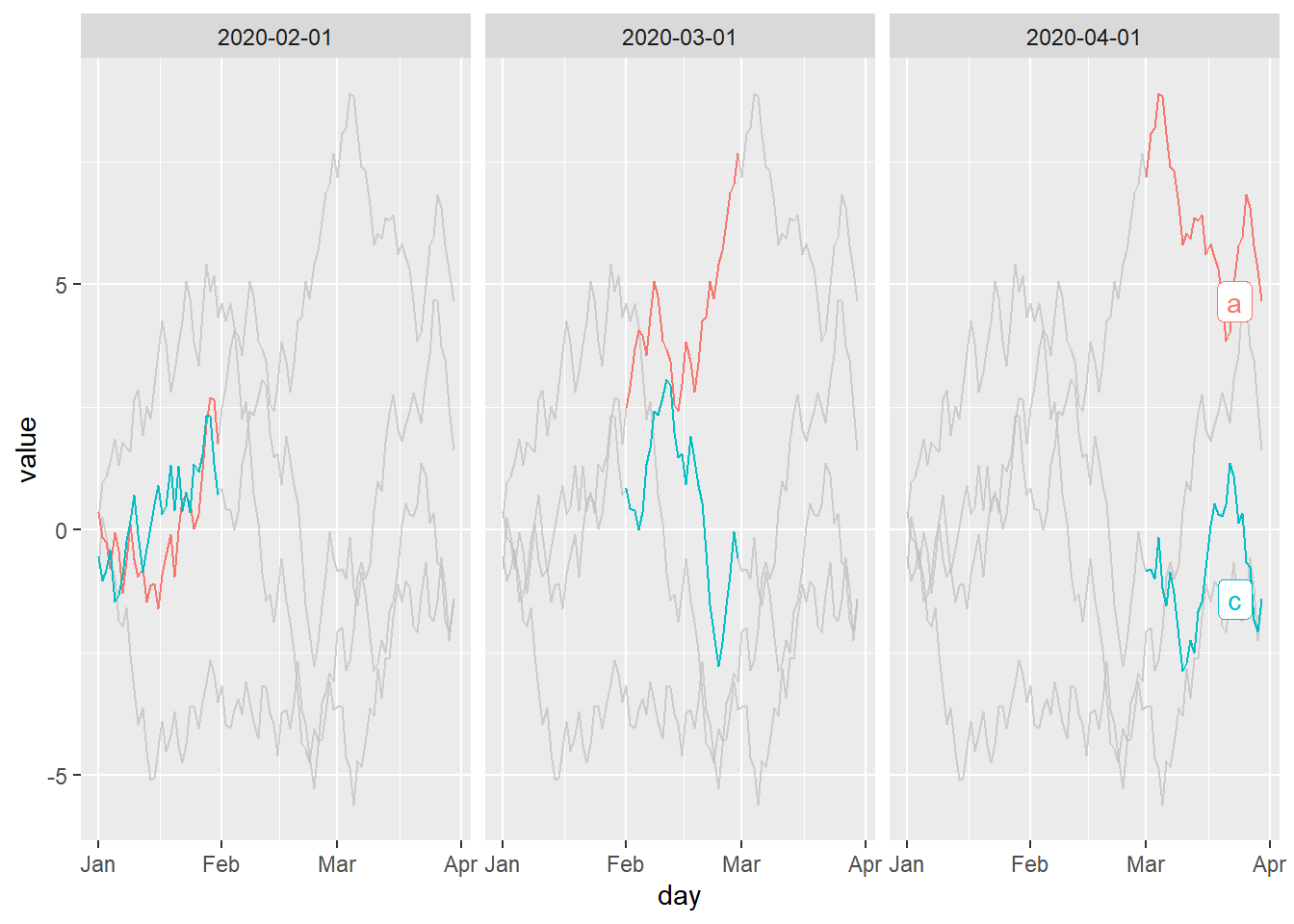But, it sometimes feels better to highlight facet by facet. For such a need, `gghighlight()` now has a new argument `calculate_per_facet`.

``````p +
gghighlight(mean(value) > 0,
calculate_per_facet = TRUE,
keep_scales = TRUE)``````
``label_key: id``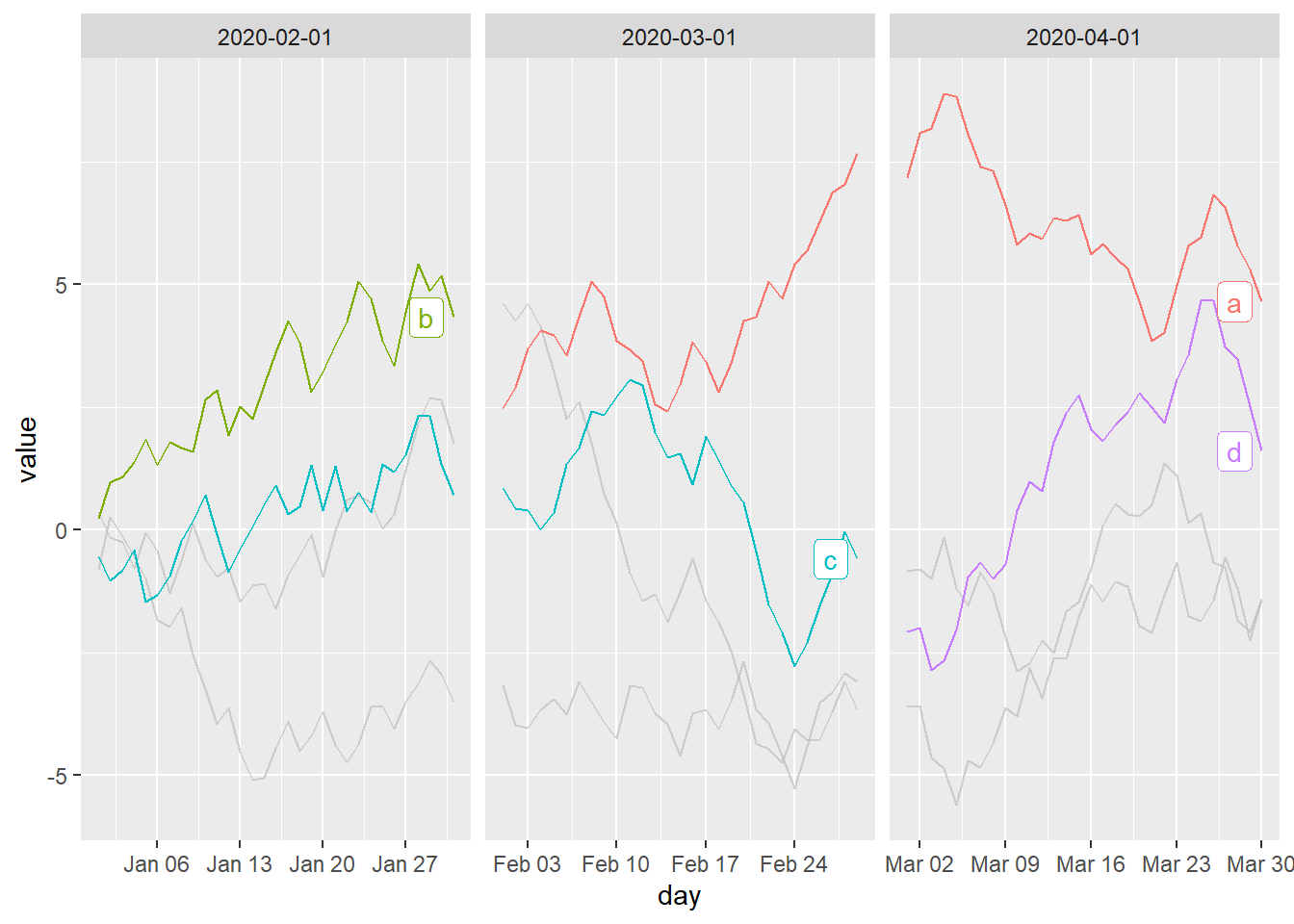Note that, as a general rule, only the layers before adding `gghighlight()` are modified. So, if you add facet_*() after adding gghighlight(), this option doesn’t work (though this behaviour might also be useful in some cases).

``````ggplot(d) +
geom_line(aes(day, value, colour = id)) +
gghighlight(mean(value) > 0,
calculate_per_facet = TRUE,
keep_scales = TRUE) +
facet_wrap(~ month, scales = "free_x")``````
``label_key: id``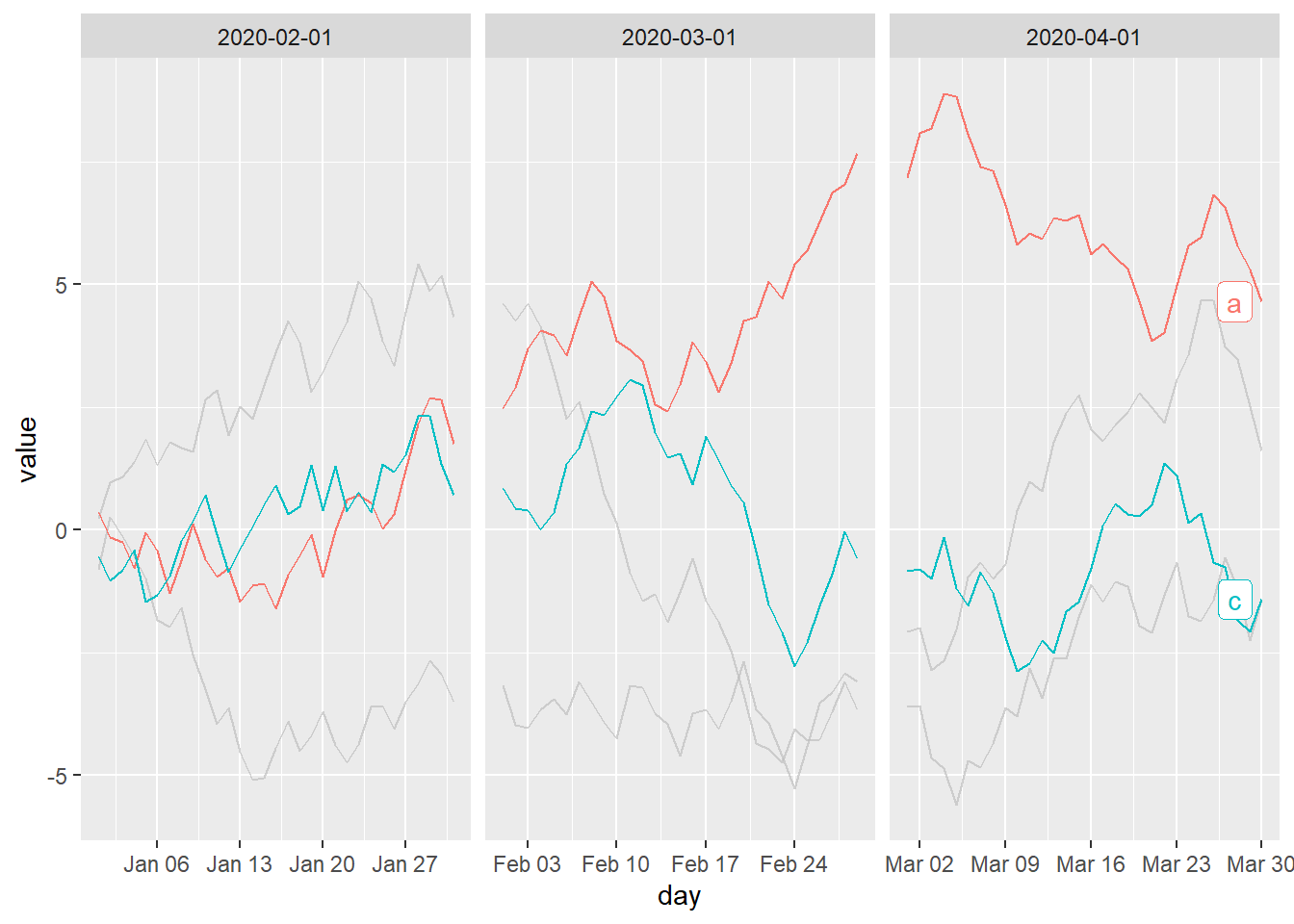## `unhighlighted_params`

`gghighlight()` now allows users to override the parameters of unhighlighted data via `unhighlighted_params`. This idea was suggested by @ClausWilke.

To illustrate the original motivation, let’s use an example on the ggridges’ vignette. gghighlight can highlight almost any Geoms, but it doesn’t mean it can “unhighlight” arbitrary colour aesthetics automatically. In some cases, you need to unhighlight them manually. For example, `geom_density_ridges()` has `point_colour`.

``````library(ggplot2)
library(gghighlight)
library(ggridges)

p <- ggplot(Aus_athletes, aes(x = height, y = sport, color = sex, point_color = sex, fill = sex)) +
geom_density_ridges(
jittered_points = TRUE, scale = .95, rel_min_height = .01,
point_shape = "|", point_size = 3, size = 0.25,
position = position_points_jitter(height = 0)
) +
scale_y_discrete(expand = c(0, 0)) +
scale_x_continuous(expand = c(0, 0), name = "height [cm]") +
scale_fill_manual(values = c("#D55E0050", "#0072B250"), labels = c("female", "male")) +
scale_color_manual(values = c("#D55E00", "#0072B2"), guide = "none") +
scale_discrete_manual("point_color", values = c("#D55E00", "#0072B2"), guide = "none") +
coord_cartesian(clip = "off") +
guides(fill = guide_legend(
override.aes = list(
fill = c("#D55E00A0", "#0072B2A0"),
color = NA, point_color = NA)
)
) +
ggtitle("Height in Australian athletes") +
theme_ridges(center = TRUE)

p +
gghighlight(sd(height) < 5.5)``````
``Picking joint bandwidth of 2.8``
``Picking joint bandwidth of 2.23``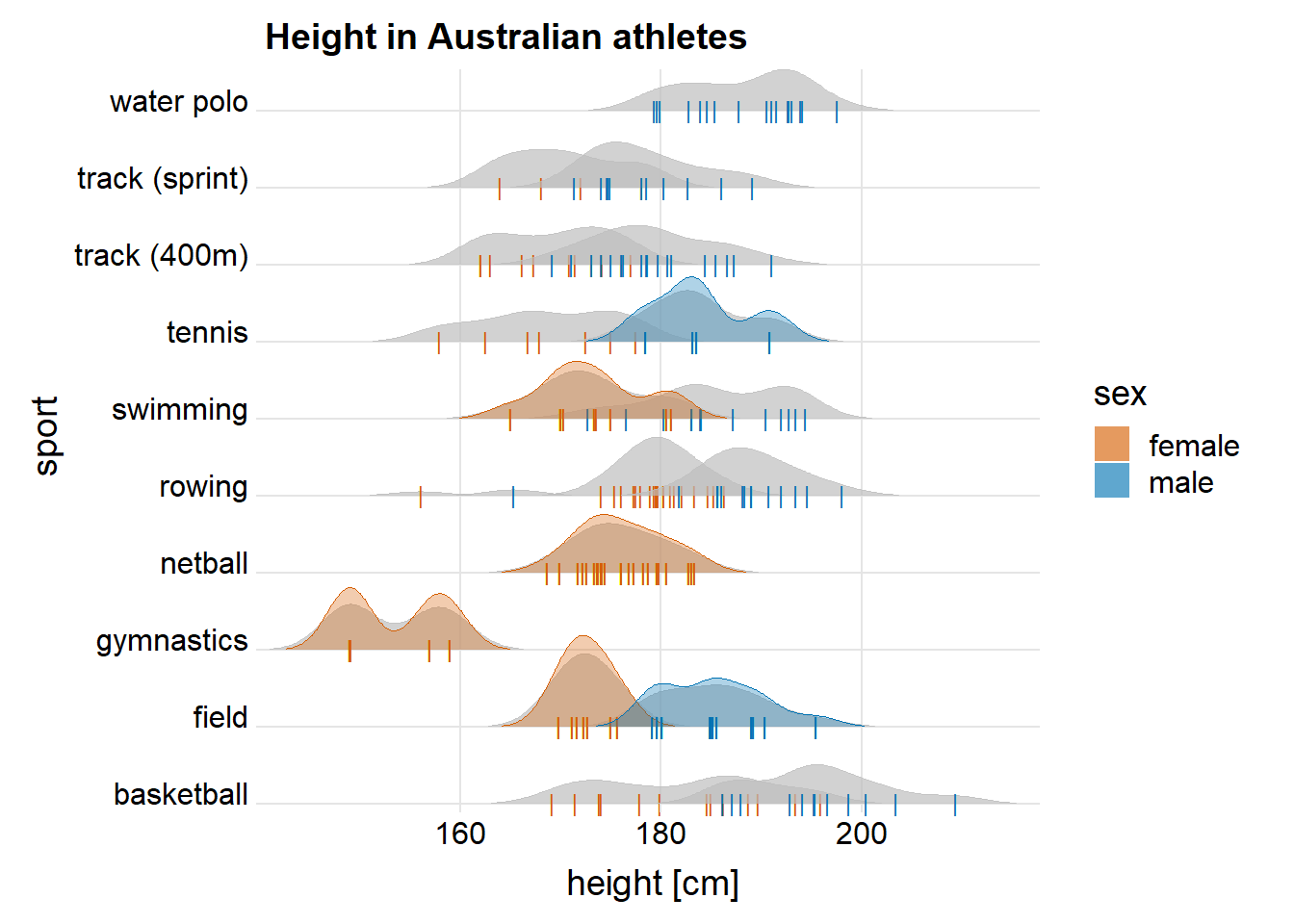You should notice that these vertical lines still have their colours. To grey them out, we can specify `point_colour = "grey80"` on `unhighlighted_params` (Be careful, `point_color` doesn’t work…).

``````p +
gghighlight(sd(height) < 5.5,
unhighlighted_params = list(point_colour = "grey80"))``````
``Picking joint bandwidth of 2.8``
``Picking joint bandwidth of 2.23``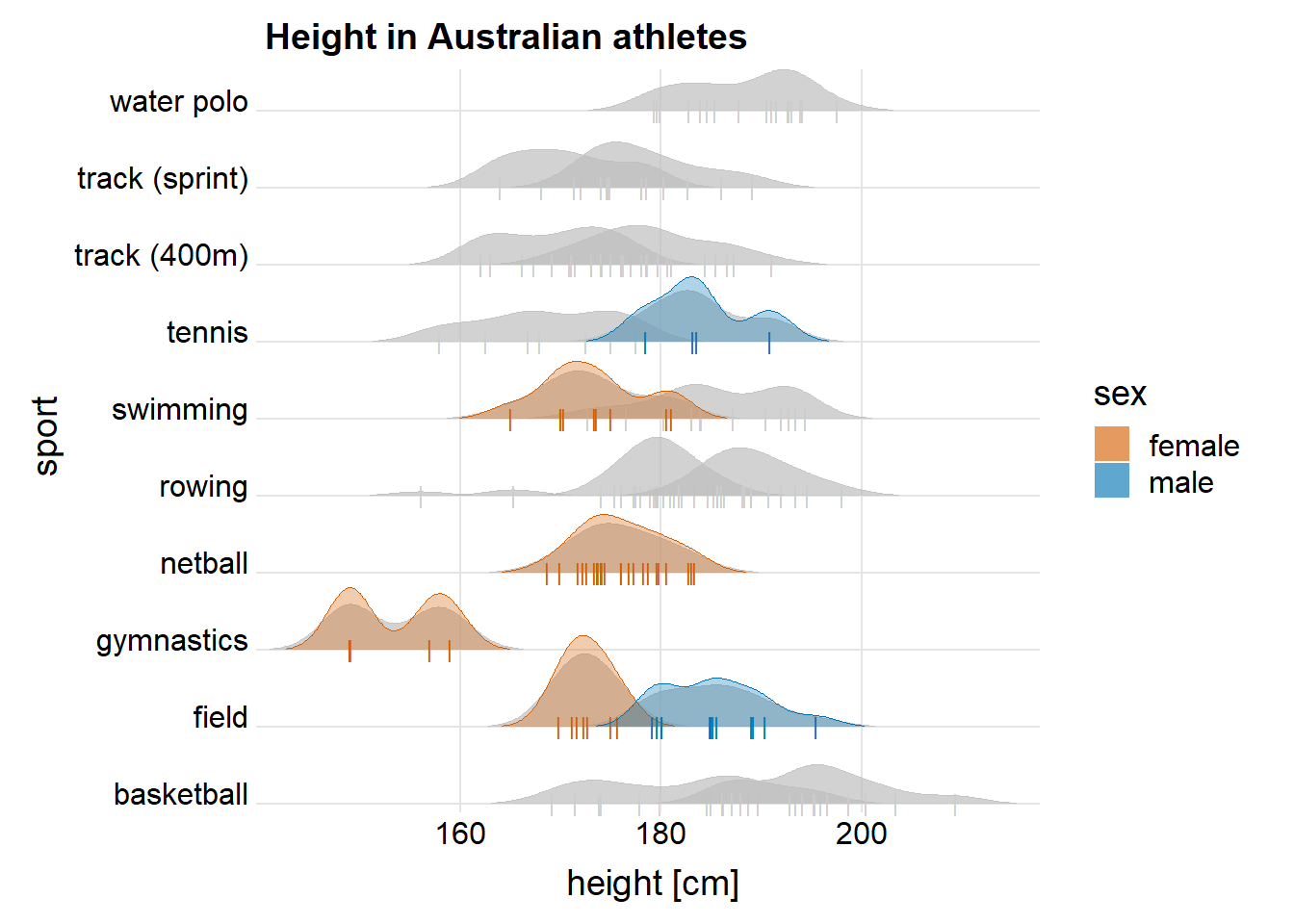`unhighlighted_params` is also useful when you want more significant difference between the highlighted data and unhighligted ones. In the following example, `size` and `colour` are set differently.

``````set.seed(2)
d <- purrr::map_dfr(
letters,
~ data.frame(
idx = 1:400,
value = cumsum(runif(400, -1, 1)),
type = .,
flag = sample(c(TRUE, FALSE), size = 400, replace = TRUE),
stringsAsFactors = FALSE
)
)

ggplot(d) +
geom_line(aes(idx, value, colour = type), size = 5) +
gghighlight(max(value) > 19,
unhighlighted_params = list(size = 1, colour = alpha("pink", 0.4)))``````
``````size aesthetic has been deprecated for use with lines as of ggplot2 3.4.0